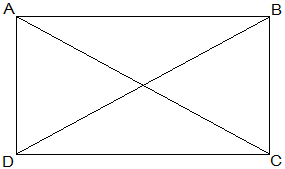Guru

# If the diagonals of a parallelogram are equal, then show that it is a rectangle. Q.2

• 0

What is the best way for solving the question from class 9th ncert math of Quadrilaterals chapter of Ncert of exercise 8.1of math. What is the best way for solving this question please guide me the best way for solving this question. If the diagonals of a parallelogram are equal, then show that it is a rectangle.

Share

1.Given that,

AC = BD

To show that, ABCD is a rectangle if the diagonals of a parallelogram are equal

To show ABCD is a rectangle we have to prove that one of its interior angles is right angled.

Proof,

AB = BA (Common)

BC = AD (Opposite sides of a parallelogram are equal)

AC = BD (Given)

Therefore, ΔABC ≅ ΔBAD [SSS congruency]

∠A = ∠B [Corresponding parts of Congruent Triangles]

also,

∠A+∠B = 180° (Sum of the angles on the same side of the transversal)

⇒ 2∠A = 180°

⇒ ∠A = 90° = ∠B

Therefore, ABCD is a rectangle.

Hence Proved.

• 0